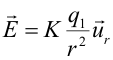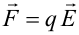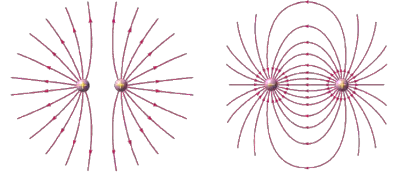# What Is an Electric Field? Concept, Definition and FormulaIn physics, the electric field is a force field generated in space by the presence of one or more electric charges or a time-varying magnetic field. Together with the magnetic field, it constitutes the electromagnetic field, responsible for electromagnetic interaction.

Introduced by Michael Faraday, the electric field propagates at the speed of light and exerts a force on any electrically charged object.

If it is generated only by the stationary distribution of space charge, the electric field is called the electrostatic field and it is conservative.

An instrument called an electroscope is used to measure the electrification of a body.

Static electricity also generates an electric field. In this case, it is a static electric field that is the force field generated by the repulsion and attraction of positive and negative charges fixed in space.

## Electric Field Formula

The formula for the electric field (E) at a point P generated by a point electric charge q1 is:where:

• E is the vector of the electric field intensity that indicates the magnitude and direction of the field.
• q1 is the value of the measured load. The unit of electric charge in the international system of units is the Coulomb.
• K is the electrostatic constant that depends on the medium. the value of k in vacuum is 9 · 10⁹ Nm² / C.
• r is the distance from the charge to the point where the electric field is measured.
• ur is a unit vector that goes from the source charge towards the point P.

In the international system of measurement units it is measured in newtons per coulomb (N / C), or in volts per meter (V / m).

The electric field can also be calculated for a continuous charge distribution. In this case, it is calculated by making the vector sum of the fields generated by each electric charge. However, because it is difficult to calculate, it is easier to use Gauss's law.

Once the vector of the electric field is known, the force that this field exerts on a witness charge located at P can be calculated:Therefore, if the control charge is positive, the force exerted by the field will be parallel to the field. On the contrary, if the charge located at a certain point is negative, the force will be in the opposite direction to the field.

## What Are the Lines of an Electric Field?

The field lines are imaginary lines that describe the trajectory that a positive charge located freely at a point would describe to pass from this point to another within an electric field.## Coulomb's Law

From these experimental evidences of electric fields, Charles Augustin de Coulomb formulated Coulomb's law. Coulomb's law quantifies the electrical force of attraction and repulsion of electrical charges located at a certain distance.

From this law it can be stated that an electrically charged body produces a field in the surrounding space such that, if a point charge is introduced, it is affected by the effect of a force, called the Coulomb force.

Author:

Published: October 6, 2021
Last review: September 6, 2021